# AP Board 7th Class Maths Solutions Chapter 2 Fractions, Decimals and Rational Numbers InText Questions

SCERT AP 7th Class Maths Solutions Pdf Chapter 2 Fractions, Decimals and Rational Numbers InText Questions and Answers.

## AP State Syllabus 7th Class Maths Solutions 2nd Lesson Fractions, Decimals and Rational Numbers InText Questions

Let’s Explore [Page No. 24]

Question 1.
Here are equivalent fractions containing 1 to 9 digits only once.
Eg: $$\frac{2}{6}=\frac{3}{9}=\frac{58}{174}$$ or
$$\frac{2}{4}=\frac{3}{6}=\frac{79}{158}$$
Can you write some more?
$$\frac{3}{21}=\frac{8}{56}=\frac{7}{49}$$
$$\frac{3}{27}=\frac{6}{54}=\frac{9}{81}$$
and so on.

Let’s Think [Page No. 24]

We know that different operations with the same pair of fractions usually give different answers. Observe the following calculations which are some interesting exceptions in fractions.
(1) 11/6 + 11/5 = 11/6 × 11/5
(2) 169/30 + 13/15 = 169/30 × 13/15

Lets Do Activity [Page No. 241]

Question 1.
Make two dice with card board or wood. Paste colour chart papel- to the all faces of the each dice. Write any three like fractions and three unlike fractions on faces of each dice. Now in a group each time two students throw the dice who are sitting opposite to each other. Write the come up fraction on the dice In the given table and do the four fundamental operations with those two fractions. Submit the Ailed table to your teacher.
Let the fractions be $$\frac{1}{3}, \frac{2}{3}, \frac{5}{3}$$ and $$\frac{1}{2}, 1 \frac{3}{4}, \frac{5}{6}$$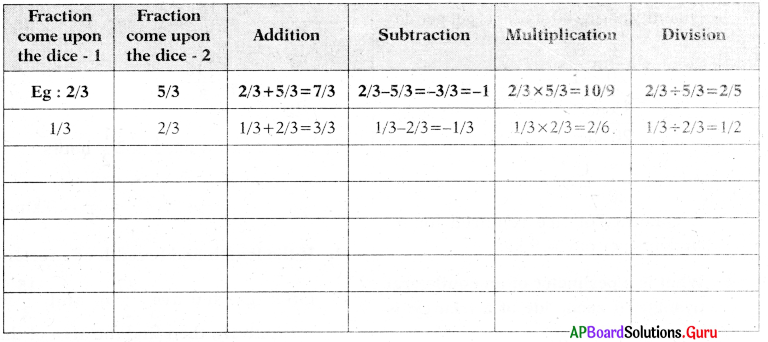[Page No. 27]

Observe the following table and fill in the blanks.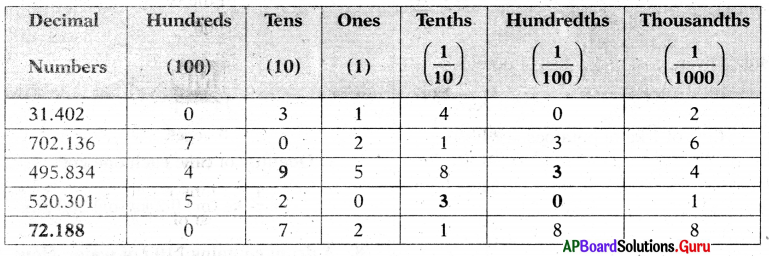Check Your Progress [Page No. 28]

Find the product:
Question 1.
32.5 × 8
32.5 × 8
= $$\frac{325}{10}$$ × 8
= $$\frac{2600}{10}$$
∴ 32.5 × 8 = 260

Question 2.
94.62 × 7
94.62 × 7
= $$\frac{9462}{100} \times \frac{7}{1}$$
= $$\frac{66234}{100}$$
∴ 94.62 × 7 = 662.34

Question 3.
109.761 × 31
109.761 × 31
= $$\frac{109761}{1000}$$ × 31
= $$\frac{3402591}{1000}$$
∴ 109.761 × 31 = 3402.591

Question 4.
61 × 2.39
61 × 2.39
= 61 × $$\frac{239}{100}$$
= $$\frac{14579}{100}$$
∴ 61 × 2.39 = 145.79

Check Your Progress [Page No. 29]

Find the values of the following,
(i) 26.59 × 10
26.59 × 10
Number of zeroes in 10 is one. So, decimal point has to shift to one place right in the product.
∴ 26.59 × 10 = 265.9

(ii) 206.5 × 100
206.5 × 100
Number of zeroes in 100 is two. So, decimal point has to shift to two places right in the product.
∴ 206.5 × 100 = 20650

(iii) 206.5 × 1000
206.5 × 1000
Number of zeroes in 1000 is three. So, decimal point has to shift to three places right in the product.
∴ 206.5 × 100 = 206500

(iv) 10.001 × 1000
10.001 × 1000
Number of zeroes in 1000 is three. So, decimal point has to shift to three places right in the product.
∴ 10.001 × 1000 = 10001

Check Your Progress [Page No. 30]

Question 1.
Find the product of the following,
(i) 69.2 × 2.5
69.2 × 2.5
= $$\frac{692}{10} \times \frac{25}{10}$$
= $$\frac{692 \times 25}{10 \times 10}$$
= $$\frac{17300}{100}$$
∴ 69.2 × 2.5 = 173

(ii) 20.61 × 3.09
20.61 × 3.09
$$\frac{2061}{100} \times \frac{309}{100}=\frac{636849}{10000}$$
∴ 20.61 × 3.09 = 63.6849

(iii) 658.321 × 43.2
658.321 × 43.2
= $$\frac{658321}{1000} \times \frac{432}{10}$$
= $$\frac{658321 \times 432}{1000 \times 10}$$
= $$\frac{284394672}{10000}$$
∴ 658.321 × 43.2 = 28439.4672

(iv) 206.005 × 0.07
206.005 × 0.07
= $$\frac{206005}{1000} \times \frac{7}{100}$$
= $$\frac{206005 \times 7}{1000 \times 100}$$
= $$\frac{1442035}{100000}$$
∴ 206.005 × 0.07 = 14.42035Let’s Explore [Page No. 30]

Question 1.
Observe the figure. Fill the blue boxes with suitable decimal numbers.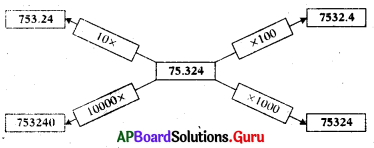→ I am a decimal number, who is half of one fourth of 100. Who am I?
12.5

[Page No. 32]

Fill the following blanks:

 169.28 ÷ 10 = 16.928 525.9 ÷ 10 = _______ 169.28  ÷ 100 = 1.6928 525.9  ÷ 100 = ______ 169.28 ÷ 1000 = _________ 525.9  ÷ 1000 = ______

 169.28 ÷ 10 = 16.928 525.9 ÷ 10 = 52.59 169.28  ÷ 100 = 1.6928 525.9  ÷ 100 = 5.259 169.28 ÷ 1000 = 0.16928 525.9  ÷ 1000 = 0.5259

Check Your Progress [Page No. 32]

Question 1.
Find the following.
(i) 81.5 ÷ 10
81.5 ÷ 10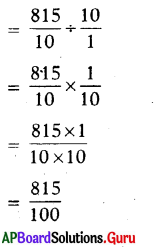∴ 81.5 ÷ 10 = 8.15

(ii) 4901.2 ÷ 100
4901.2 ÷ 100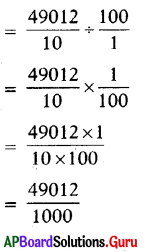∴ 4901.2 ÷ 100 = 49.012

(iii) 7301.3 ÷ 1000
7301.3 ÷ 1000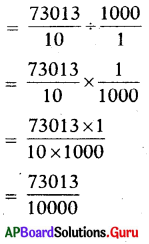∴ 7301.3 ÷ 1000 = 7.3013

(iv) 1.2 ÷ 100
1.2 ÷ 100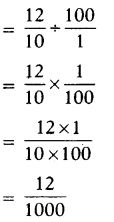∴ 1.2 ÷ 100 = 0.012

[Page No. 33]

Question 2.
Find the following :
(i) 69.4 ÷ 2
69.4 ÷ 2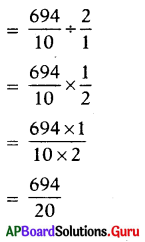∴ 69.4 ÷ 2 = 34.7

(ii) 56.32 ÷ 8
56.32 ÷ 8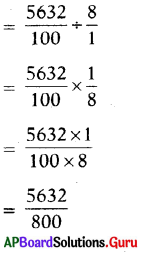∴ 56.32 ÷ 8 = 7.04

(iii) -6.5 ÷ 4
6.5 ÷ 4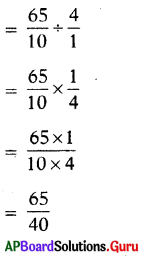∴ 6.5 ÷ 4 = 1.625

(iv) 108.7 ÷ 5
108.7 ÷ 5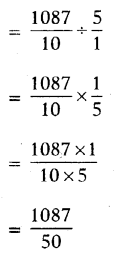∴ 108.7 ÷ 5 = 21.74

Check Your Progress [Page No. 35]

Solve the following :
(i) 0.45 ÷ 0.9
0.45 ÷ 0.9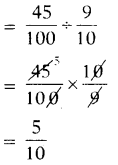∴ 0.45 ÷ 0.9 = 0.5

(ii) 2.125 ÷ 0.05
2.125 ÷ 0.05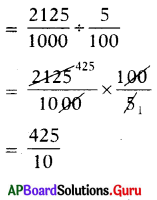∴ 2.125 ÷ 0.05 = 42.5

(iii) 94.3 ÷ 0.004
94.3 ÷ 0.004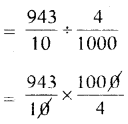= 943 × 25
∴ 94.3 ÷ 0.004 – 23575

iv) 10.25 ÷ 0.2
10.25 ÷ 0.2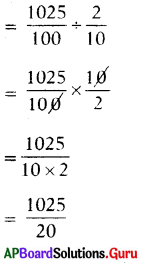∴ 10.25 ÷ 0.2 = 51.25Examples:

Question 1.
In the school out of 180 students, of the students are boys. Find the number of girls in the school?
Number of students in the school = 180
Part oL the boys in the school = $$\frac{4}{9}$$
Number of boys = $$\frac{4}{9}$$ of 180
= $$\frac{4}{9}$$ × 180 = 80
∴ Number of girls = 180 – 80 = 100

Question 2.
if the cost of 22$$\frac{1}{2}$$ kg. of apples (a box) in a whole sale market is ₹ 1170, then find the cost of 5 kg. of apples.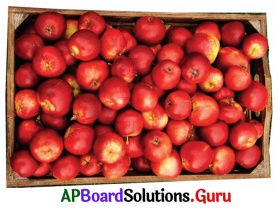Cost 0f 22$$\frac{1}{2}$$kg. of apples = ₹ 1170
Cost of 1 kg. ofapple = ₹ 1170 ÷ 22$$\frac{1}{2}$$
= ₹ 1170 × $$\frac{45}{2}$$
= ₹1170 × $$\frac{45}{2}$$ = ₹ 52
= 1170 × $$\frac{2}{45}$$

∴ Cost of 5kg. óf apples = 5 × ₹ 52
= ₹ 260

Question 3.
If one side of a square is 3.8 cm, then find its perimeter.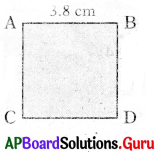Number of sides to a square = 4
Side of a square = 3.8 cm
Each side of a square is equal.

Perime ret of a square = 4 × side
= 4 × 3.8
= 15.2 cm

Question 4.
Find :
(i) 239.27 × 10
239.27 × 10 (number of zeroes in 10 is one. So, decimal point has to shift to one place right in the product).
∴ 239.27 × 10 = 2392.7

(ii) 5.305 × 100
5.305 × 100 = 530.5

(iii) 23.1 × 1000
23.1 × 1000 = 23100.0 = 23100

Question 5.
Bindu went to vegetable market with her mother to buy 3.5 kg Onions. If the cost of Onions is ₹ 18.50 per kg., then find the cost of 3.5 kg of Onions.
Cost of 1 kg. Onions = ₹ 18.50
Cost of 3.5 kg. of Onions = ₹ 18.50 × 3.5 = 64.750
Cost of 3.5 kg. of Onions = ₹ 64.75

Step 1 : Multiply whole numbers ignoring decimals
35 × 1850 = 64750.

Step 2: As there are total 2 + 1 = 3 decimals, put decimal point after three digits from the right most to the product.
So, 3.5 × 18.50 = 64.750.

Question 6.
Madhuri is studying 7th class in Visakhapatnam. Her school teachers organised a tour to Araku valley by bus. Bus covered a distance of 98.5 km. in 2.5 hours. If the bus is travelled at the same speed in the journey, then find the distance travelled in 1 hour.
Distance travelled by bus = 98.5 km. Time taken to travel this distance = 2.5 hours.
∴ Distance travelled by bus in 1 hour = 98.5 ÷ 2.5
= $$\frac{985}{25}$$ = 39.4 km.
∴ Bus travelled in 1 hour = 39.4 km.Practice Questions: [Page No. 39]

Question 1.
15, 27, 39, 51, 63,……………
(a) 85
(b) 75
(c) 65
(d) 73
(b) 75

Explanation:
15, 27, 39, 51, 63,…………..
(13 × 1 + 2), (13 × 2 + 1), (13 × 3 + 0), (13 × 4 – 1), (13 × 5 – 2), ………………….
So, next number is (13 × 6 – 3)
= 78 – 3 = 75

Question 2.
2, 5, 10, 17, 26, 37, …………….
(a) 48
(b) 75
(c) 50
(d) 73
(c) 50

Explanation:
2, 5, 10, 17, 26, 37, ……….
(1 × 1 + 1), (2 × 2 + 1), (3 × 3 + 1), (4 × 4 + 1), (5 × 5 + 1), (6 × 6 + 1), ………….
So, next number is (7 × 7 + 1) = 50

Question 3.
1, 6, 16, 31, 51, 76 ……………..
(a) 95
(b) 86
(c) 91
(d) 96
No option.

Explanation:
1, 6, 16, 31, 51, 76, …………..
1, (1 + 5), (6 + 10), (16 + 15), (31 + 20), (51 + 25), (76 + 30), ……………
∴ So, next number is (76 + 30) = 106.

Question 4.
13, 14, 16, 20, 28, 44, …………..
(a) 76
(b) 75
(c) 87
(d) 73
(a) 76

Explanation:
13, 14, 16, 20, 28, 44, …………
(12 + 20), (12 + 21), (12 + 22), (12 + 23), (12 + 24), (12 + 25),
So, next number is 12 + 26 = 12 + 64 = 76

Question 5.
28, 25, 30, 27, 32, 29, ………….
(a) 26
(b) 24
(c) 34
(d) 32
(c) 34

Explanation:
28, 25, 30, 27, 32, 29, …………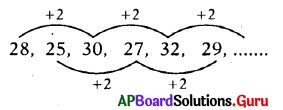So, next number is 32 + 2 = 34Question 6.
3, -6, 12, -24, 48, -96, ………..
(a) 192
(b)- 102
(c)- 192
(d) 106
(a) 192

Explanation:
3, -6, 12, -24, 48, -96, …………
3, (3 × -2), (-6 × -2), (12 × -2), (-24 × -2), (48 × -2)…………..
So, next number is (-96 × – 2) = 192.

Question 7.
1, 2, 6, 24, 120, 720, ……………….
(a) 920
(b)5040
(c) 1040
(d)4320
(b)5040

Explanation:
1, 2, 6, 24, 120, 720, …………………
1, (1 × 2), (2 × 3), (6 × 4), (24 × 5), (120 × 6), ……………..
So, next number is 720 × 7 = 5040

Question 8.
63, 64, 67, 72, 79,……………
(a) 88
(b) 86
(c) 87
(d) 98
(a) 88

Explanation:
63, 64, 67, 72, 79, ………..
63, (63 + 1), (64 + 3), (67 + 5), (72 + 7),….
So, next number is (79 + 9) = 88

Question 9.
9, 10, 22, 69, 280, …………..
(a) 1205
(b) 1425
(c) 1400
(d)1405
(d)1405

Explanation:
9, 10, 22, 69, 280, ………….
9, (9 × 1 + 1), (10 × 2 + 2), (22 × 3 + 3), (69 × 4 + 4),………………
So, next number is (280 × 5 + 5) = 1405

Question 10.
729, 243, 81, 27, …………….
(a) 65
(b) 18
(c) 9
(d) 73
(c) 9

Explanation:
729, 243, 81, 27 ………….
(729 ÷ 3 = 243), (243 ÷ 3 = 81), (81 ÷ 3 = 27), ……………….
So, next number is 27 ÷ 3 = 9

Question 11.
5, 15, 35, 75, 155,
(a) 215
(b) 305
(c) 315
(d) 265
(c) 315

Explanation:
5, 15, 35, 75, 155, ………..
5 × 1, 5 × 3, 5 × 7, 5 × 15, 5 × 31, …………….
5(21 – 1), 5(22 – 1), 5(23 – 1), 5(24 – 1), 5(25 – 1), ………..
So, next number is 5 x (26 – 1) = 5 × 63 = 315

Question 12.
240, 240, 120, 40, ……………., 2.
(a) 10
(b) 20
(c) 18
(d) 35
(a) 10

Explanation:
240, 240, 120, 40, ……………….,2.
240, 240 ÷ 1, 240 ÷ 2, 120 ÷ 3, 40 ÷ 4, 10 ÷ 5
So, next number is 40 ÷ 4 = $$\frac{40}{4}$$ =10

Question 13.
20, 10, 10, 20, 80, ……….
(a) 320
(b) 640
(c) 400
(d) 80
(b) 640

Explanation:
20, 10, 10, 20, 80, ……………
20, 20 × 2-1, 10 × 20, 10 × 21, 20 × 22,
So, next number is 80 × 23
= 80 × 8 = 640

Question 14.
7, 10, 8, 11, 9, 12, ………….
(a) 8
(b) 14
(c) 15
(d) 10
(d) 10

Explanation: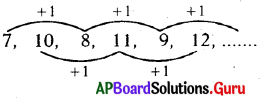So, next number is 9 + 1 = 10.Question 15.
34, 30, 28, 24, 22, 18, ………….
(a) 16
(b) 14
(c) 20
(d) 15
(a) 16

Explanation:
34, 30, 28, 24, 22, 18, ……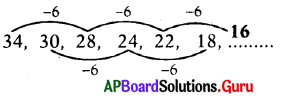So, next number is (22 – 6) = 16

Number Series – 2

Question 1.
Adding or subtract of natural numbers:
Eg: 6, 7, 9, 12, 16, 21,…
(a) 21
(b) 25
(c) 27
(d) 28
(c) 27

Explanation:
(6 + 1), (7 + 2,), (9 + 3), (12 + 4), (16 + 5)
so, next number is (21 + 6) = 27

Question 2.
Eg: 10, 20, 40, 70, 110, …
(a) 160
(b) 180
(c) 150
(d) 210
(a) 160

Explanation:
(10 + 10,), (20 + 20), (40 + 30), (70 + 40)
so, next number is (110 + 50) = 160

Question 3.
Eg: 27, 26, 23, 18, 11, …
(a) 4
(b) 2
(c) 9
(d) 5
(b) 2

Explanation:
(27 – 1), (26 – 3), (23 – 5) (18 – 7)
so, next number is (11 – 9) = 2

Question 4.
Multiply with a fixed number
Eg: 5, 15, 45, 135, 405, ……..
(a) 1200
(b) 1215
(c) 850
(d) 925
(b) 1215

Explanation:
(5 × 3), (15 × 3), (45 × 3), (135 × 3),
so, next number is (405 × 3) = 1215

Question 5.
Multiply and add with same natural numbers:
Eg: 5, 6, 14, 45 ………….
(a) 184
(b) 180
(c) 176
(d) 225
(a) 184

Explanation: (5 × 1) + 1, (6 × 2) + 2, (14 × 3) +3,
so, next number is (45 × 4) + 4 = 184

Question 6.
Multiply and add with a different fixed numbers
Eg: 3, 9, 21, 45, 93 …
(a) 184
(b) 187
(c) 186
(d) 189
(b) 187

Explanation:
(3 × 2) + 3, (9 × 2) + 3, (21 × 2) + 3, (45 × 2) + 3,
so, next number is (92 x 2) +3 = 187

Question 7.
Multiply with fixed number and add different numbers:
Eg: 12, 25, 52, 107.
(a) 196
(b) 207
(c) 214
(d) 218
(d) 218

Explanation: (12 × 2) + 1, (25 × 2) + 2, (52 × 2) + 3,
so, next number is (107 × 2) + 4 = 218

Question 8.
Multiply the sequence number :
Eg: 7, 14, 42, 168, 840.
(a) 1680
(b) 5040
(c) 760
(d) 4200
(b) 5040

Explanation:
(7 × 2), (14 × 3), (42 × 4), (168 × 5)
so, next number is (840 × 6) = 5040Question 9.
Dividing with a fixed number
Eg: 256, 128, 64, 32, 16, …
(a) 8
(b) 4
(c) 16
(d) 10
(a) 8

Explanation: (256/2), (128/2), (64/2),(32/2), ……….
so, next number is (16/2) = 8

Question 10.
Multiply and divide with a different fixed number.
Eg: 12, 60, 30, 150, 75,
(a) 325
(b) 150
(c) 375
(d) 300